# Quick calculate

## Related software (5)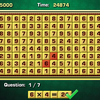## Arithmetic Game

Complete the equations in the interesting arithmetic test!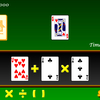## Make 24

Use the given cards to form a valid equation within 60 seconds!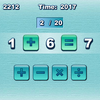## Quick Math

How quickly can you complete the equations with arithmetic symbols?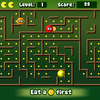## Math Man

Play Ghost Man with a mathematical twist!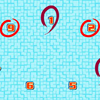## Make 15

Pick 3 numbers that add up to 15.